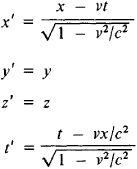# Lorentz Transformations

Also found in: Dictionary.
The following article is from The Great Soviet Encyclopedia (1979). It might be outdated or ideologically biased.

## Lorentz Transformations

in the special theory of relativity, transformations of the coordinates and time of an event on changing from one inertial frame of reference to another. These transformations were derived in 1904 by H. A. Lorentz as transformations for which the equations of classical microscopic electrodynamics (the Lorentz-Maxwell equations) retain their form. In 1905, A. Einstein derived them by proceeding from two postulates that formed the basis of the special theory of relativity: the equivalence of all inertial frames of reference and the independence of the velocity of light in a vacuum from the motion of the source of light.

Let us examine a particular case of two inertial reference frames Σ and Σ’ with axes x and x’ lying on the same straight line and with the other correspondingly parallel axes (y and y’, z and z’). If the system Σ’ moves with respect to Σ at a constant velocity v in the direction of the x-axis, then the Lorentz transformations for the transition from Σ to Σ’ have the formwhere c is the velocity of light in a vacuum (the coordinates with primes refer to system Σ’, and the coordinates without primes, to Σ).

The Lorentz transformations lead to a number of important consequences, such as the law of addition of velocities in the theory of relativity and the dependence of the linear dimensions of bodies and time intervals on the selected frame of reference. For rates of motion that are small in comparison with the velocity of light (v « c), the Lorentz transformations become Galilean transformations, which are valid in classical Newtonian mechanics.

G. A. ZISMAN

The Great Soviet Encyclopedia, 3rd Edition (1970-1979). © 2010 The Gale Group, Inc. All rights reserved.
References in periodicals archive ?
One can see from (17) that in the flat limit h; g [right arrow] 0 the above transformations become the Lorentz transformations, as expected.
While, if [eta] [not equal to] 1 the Fresnel's drag effect in the rest frame of the medium ([summation]) cancels the effect of the genuine Lorentz transformation to a moving frame ([summation]').
The continuous Lorentz transformations (including the spatial rotations) operate on the "heavenly sphere" , i.
The analog of Lorentz transformations in C-spaces which transform a polyvector X into another poly-vector X' is given by
[1-5], the Dirac 4 x 4 [gamma]-matrices ([[gamma].sup.[mu]]) are usually presented as objects that undergo a transformation during a Lorentz transformation of the Dirac [6.7] equation.
In addition, one can show  that Lorentz transformations (10) can be extended to special events, such as intersections of particle trajectories, involving massive noninteracting particles, for example, our steel ball and mirrors.
By Liouville's theorem C(1,3) is (up to removable discontinuities) the set of transformations generated by the translations, the Lorentz transformations, the conformal inversion, and the positive dilatations.
The current consensus is that SR is a proven theory and beyond doubt; yet what is actually proven is that the Lorentz transformations work to describe certain high-velocity physical phenomena, when applied to the correct Cosmic frame.
Using special Lorentz transformation for space-time and charge density-current density four-vectors , we derive for the segments 1 and 3:
This quadratic form is interpreted in Physics as the metric tensor of Minkovski spacetime, so this definition is a simply restatement of the fact that Lorentz transformations are precisely the linear transformations which are also isometries of Minkovski spacetime.
The operators corresponding to Lorentz transformations ([M.sub.[mu]]v]), translations ([P.sub.[mu]]), dilatations (D) and special conformal transformations ([K.sub.[mu]]) satisfy the following commutation relations
He begins with special relativity, including Lorentz transformations and the Doppler shift.

Site: Follow: Share:
Open / Close